Courses
Courses for Kids
Free study material
Free LIVE classes
More

# Arithmetic Patterns: Fun Way to Identify Different Patterns in a Set of NumbersLIVE
Join Vedantu’s FREE Mastercalss

Are you excited to know what an arithmetic pattern means and how to identify different arithmetic patterns? Yes? Okay! Let’s first learn the meaning of a pattern with examples before knowing about arithmetic patterns.

## What Are Patterns?

Patterns are repeated arrangements of numbers, objects, lines, colours, shapes, etc. in a logical way.

Look at the picture below to understand patterns in a better way: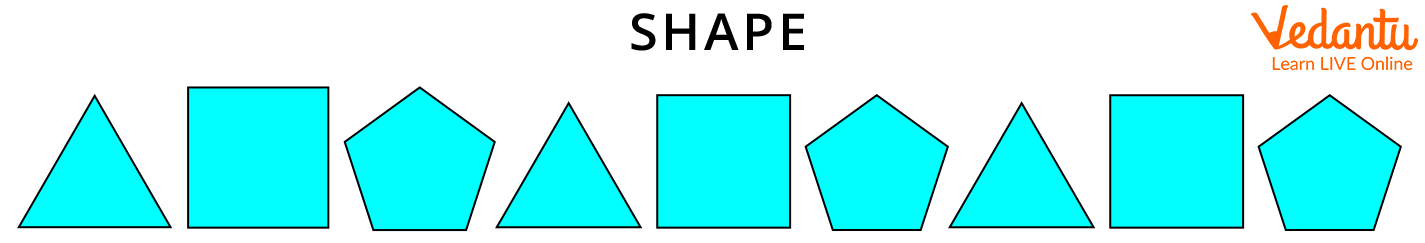Shape pattern

In the above picture, the pattern followed is:

Triangle – SquarePentagon, Triangle – Square – Pentagon, Triangle – Square – Pentagon.

Here, all shapes like triangle, square, and pentagon are exactly the same.

## Arithmetic Pattern or Algebraic Pattern

Do you want to know the other name of the arithmetic pattern?

Yes!

The other name of the arithmetic pattern is “algebraic pattern.”

Now, let’s learn the meaning of arithmetic pattern or algebraic pattern.

## Arithmetic Pattern Meaning

Arithmetic pattern or algebraic pattern is a set of numbers that are arranged in order.

For example:

0, 5, 10, 15, 20, 25

The above set of numbers follows some definite pattern.

Are you excited to know “What pattern it follows”? Let’s learn about the pattern.

Here, we used skip counting by 5 to get the next number.

In other words, we get numbers in pattern by adding ‘5’ to get the next number.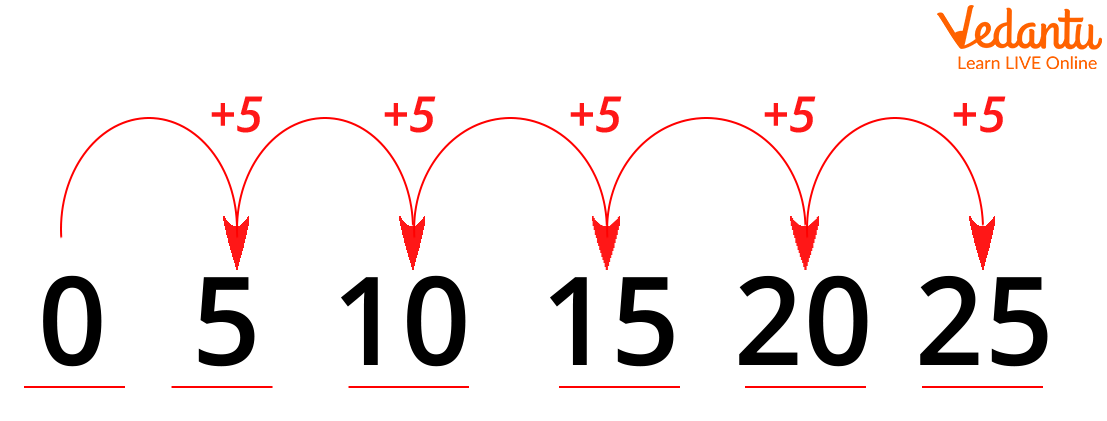Skip counting by 5

Similarly, a different set of numbers follows a different pattern. Let's learn to identify what different patterns a set of numbers can follow with examples.

## Arithmetic Patterns with Examples

Let’s learn different arithmetic patterns with examples:

### 1. Increasing Sequence

In increasing sequence, numbers in a pattern go on increasing. Suppose, you have one football. Now, if your mother gives you two more footballs, it will become three. Again, you received two more footballs from your brother. Now, the total number of footballs you have is 5 ( 1 + 2 + 2). Here, we have observed that the total number of footballs is increasing with each time you are receiving new footballs.

Look at the below picture to understand the increasing sequence pattern in a better way.Increasing pattern

### 2. Decreasing Sequence or Decreasing Pattern

In decreasing sequence, the number in a pattern goes on decreasing. Suppose there are 18 mangoes in the basket. You give three mangoes to each of your friends in the class. The number of mangoes goes on decreasing in the basket each time you give three mangoes to your friend.

Look at the below pattern to understand the decreasing pattern in a better way.

The decreasing pattern is as follows: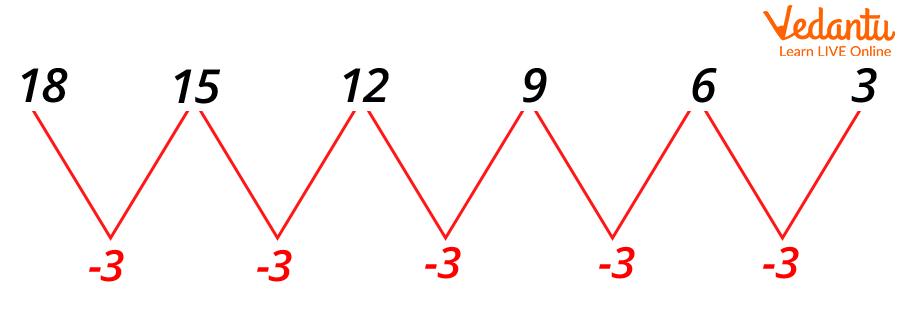Decreasing pattern

In the pattern 18, 15, 12, 9, 6, 3, we have subtracted ‘3’ each time from the previous number to get the next number.

### 3. Growing Pattern

In a growing pattern, the number in a pattern increases rapidly. Here, we multiply the previous number with the same value each time to get the next number.

Look at the below picture to understand the growing pattern in a better way.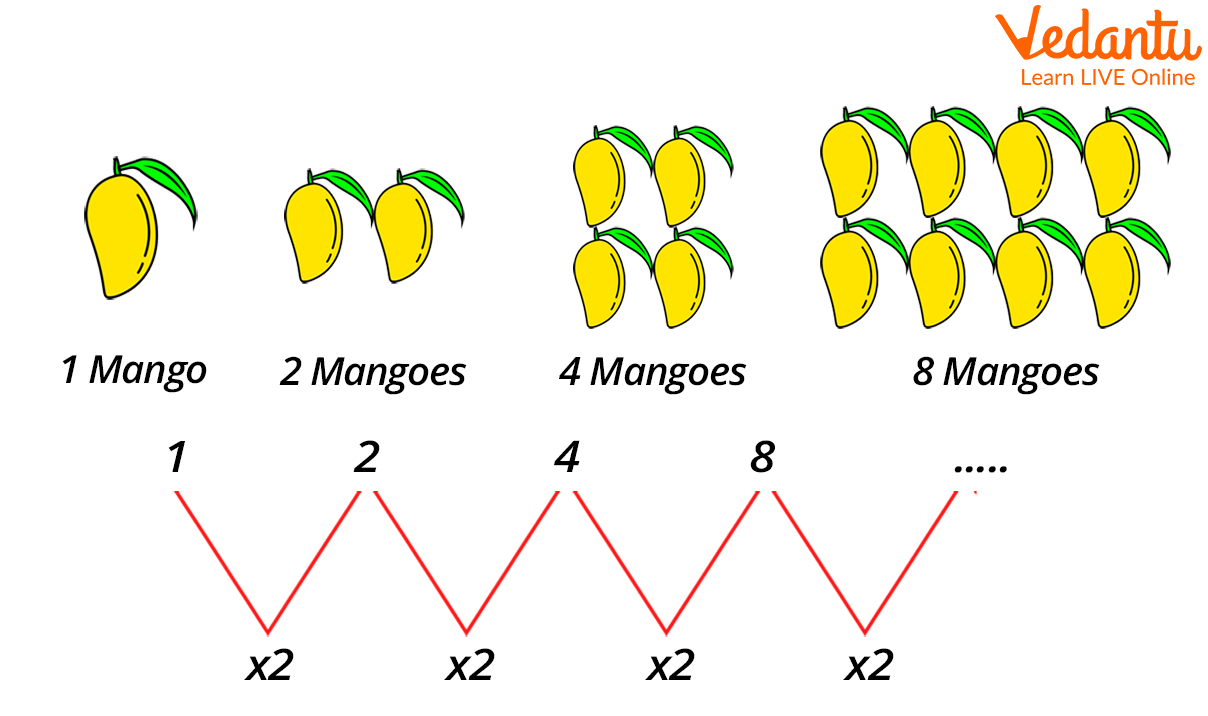Growing pattern

Here,

The pattern 1, 2, 4, 8….. is formed by multiplying two each time.

### 4. Triangular Pattern

In a triangular pattern, we add another row of dots and count all the dots to find the next number in a given pattern. Let’s understand with an example: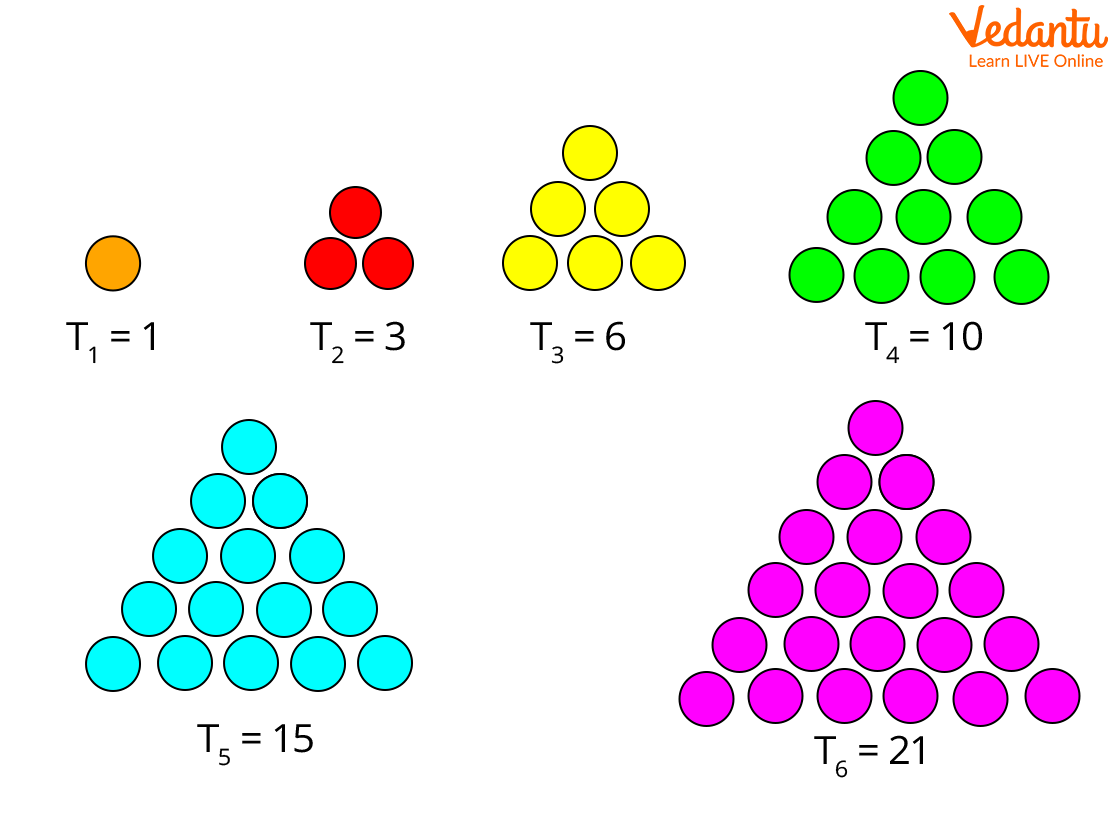Triangular pattern

Here, the first triangle has only one dot.

In the second triangle, another row is added with 2 extra dots, making 1 + 2 = 3.

In the third triangle, another row is added with 3 extra dots, making 1 + 2 + 3 = 6.

In the fourth triangle, another row is added with 4 extra dots, making 1 + 2 + 3 + 4 = 10.

In the fifth triangle, another row is added with 5 extra dots, making 1 + 2 + 3 + 4 + 5 = 15.

In the sixth triangle, another row is added with 6 extra dots, making 1 + 2 + 3 + 4 + 5 + 6 = 21.

Hence, the pattern here follows: 1, 3, 6, 10, 15, 21….

## Conclusion

In short, the arithmetic pattern is the easiest sequence to learn. It creates a string of numbers by adding or subtracting a number from a common difference that relates to one another. For example, the sequence of 3, 5, 7, 9, 12, 14 has a common difference of 2 and the numbers in the sequence are increasing by adding a common difference of 2. If you found this article interesting and wish to read more of such topics, head over to our website and explore from our collection.

Last updated date: 03rd Oct 2023
Total views: 139.5k
Views today: 1.39k

## FAQs on Arithmetic Patterns: Fun Way to Identify Different Patterns in a Set of Numbers

1. What is known as the ascending pattern of numbers?

When numbers in a pattern get larger as the sequence continues, they follow an ascending pattern. In an ascending pattern, we either perform multiplication or addition.

2. What is known as the descending pattern of numbers?

When numbers in a pattern decrease as the sequence continues, they follow a descending pattern. In a descending pattern, we either perform division or subtraction.

3. What is known as a sequence in Mathematics?

In Mathematics, a sequence is an ordered list of numbers. Each number in the sequence is known as a term. For example, in the sequence: 1,3,5,7,9…, the first term is 1, the second term is 3, the third term is 5, and so on.